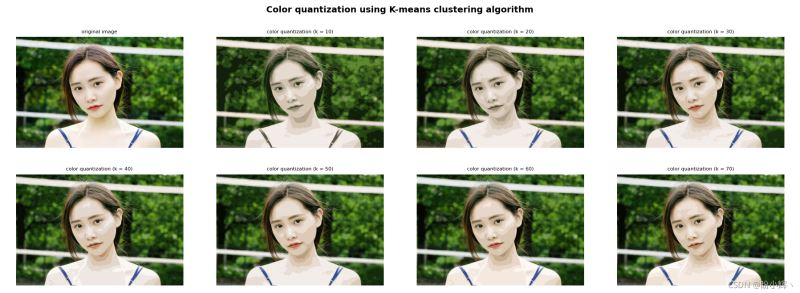﻿ Python+OpenCV实战之利用 K-Means 聚类进行色彩量化_python_脚本之家
python# Python+OpenCV实战之利用 K-Means 聚类进行色彩量化

## 前言

K-Means 聚类算法的目标是将 n 个样本划分(聚类)为 K 个簇，在博文《OpenCV与机器学习的碰撞》中，我们已经学习利用 OpenCV 提供了 cv2.kmeans() 函数实现 K-Means 聚类算法，该算法通过找到簇的中心并将输入样本分组到簇周围，同时通过简单的示例了解了 K-Means 算法的用法。在本文中，我们将学习如何利用 K-Means 聚类进行色彩量化，以减少图像中颜色数量。

## 利用 K-Means 聚类进行色彩量化

`data = np.float32(image).reshape((-1, 3))`

```img = cv2.imread('example.jpg')
```

```def color_quantization(image, k):
# 将图像转换为数据
data = np.float32(image).reshape((-1, 3))
# 算法终止条件
criteria = (cv2.TERM_CRITERIA_EPS + cv2.TERM_CRITERIA_MAX_ITER, 20, 1.0)
# K-Means 聚类
ret, label, center = cv2.kmeans(data, k, None, criteria, 10, cv2.KMEANS_RANDOM_CENTERS)
# 簇中心
center = np.uint8(center)
# 将具有 k 颜色中心的图像转换为 uint8
result = center[label.flatten()]
result = result.reshape(img.shape)
return result
color_3 = color_quantization(img, 3)
```

`color_quantization() `函数中，关键点是利用` cv2.kmeans()` 方法。最后，可以用 k 种颜色来构建图像，用它们对应的中心值替换每个像素值，程序的运行结果如下所示：### 完整代码

```import numpy as np
import cv2
from matplotlib import pyplot as plt

def show_img_with_matplotlib(color_img, title, pos):
img_RGB = color_img[:, :, ::-1]

ax = plt.subplot(2, 4, pos)
plt.imshow(img_RGB)
plt.title(title, fontsize=8)
plt.axis('off')

def color_quantization(image, k):
# 将图像转换为数据
data = np.float32(image).reshape((-1, 3))
# 算法终止条件
criteria = (cv2.TERM_CRITERIA_EPS + cv2.TERM_CRITERIA_MAX_ITER, 20, 1.0)
# K-Means 聚类
ret, label, center = cv2.kmeans(data, k, None, criteria, 10, cv2.KMEANS_RANDOM_CENTERS)
# 簇中心
center = np.uint8(center)
# 将具有 k 颜色中心的图像转换为 uint8
result = center[label.flatten()]
result = result.reshape(img.shape)
return result

fig = plt.figure(figsize=(16, 8))
plt.suptitle("Color quantization using K-means clustering algorithm", fontsize=14, fontweight='bold')
# 图片加载
show_img_with_matplotlib(img, "original image", 1)
# 使用不同 K 值进行色彩量化
for i in range(7):
color = color_quantization(img, (i+1) * 10)
show_img_with_matplotlib(color, "color quantization (k = {})".format((i+1) * 10), i+2)

plt.show()
```

## 显示色彩量化后的色彩分布

```import collections
def color_quantization(image, k):
# 将图像转换为数据
data = np.float32(image).reshape((-1, 3))
# 算法终止条件
criteria = (cv2.TERM_CRITERIA_EPS + cv2.TERM_CRITERIA_MAX_ITER, 20, 1.0)
# K-Means 聚类
ret, label, center = cv2.kmeans(data, k, None, criteria, 10, cv2.KMEANS_RANDOM_CENTERS)
# 簇中心
center = np.uint8(center)
# 将具有 k 颜色中心的图像转换为 uint8
result = center[label.flatten()]
result = result.reshape(img.shape)
# 统计分配给每个聚类中心的像素数
counter = collections.Counter(label.flatten())
print(counter)
# 计算输入图像的总像素数
total = img.shape * img.shape
# 为色彩分布图像指定宽度和高度：
desired_width = img.shape

desired_height = 70
desired_height_colors = 50
# 初始化色彩分布图像
color_distribution = np.ones((desired_height, desired_width, 3), dtype='uint8') * 255
start = 0

for key, value in counter.items():
# 归一化
value_normalized = value / total * desired_width
end = start + value_normalized
# 绘制与当前颜色对应的矩形
cv2.rectangle(color_distribution, (int(start), 0), (int(end), desired_height_colors), center[key].tolist(), -1)
start = end

return np.vstack((color_distribution, result))
```

```counter = collections.Counter(label.flatten())
```

```Counter({7: 37199, 3: 36302, 0: 29299, 5: 23987, 6: 23895, 1: 20077, 9: 19814, 8: 18427, 4: 16221, 2: 14779})
```

```np.vstack((color_distribution, result))
```Note：除了 color_quantization() 函数外，由于其他代码并未修改，因此不再另外给出。